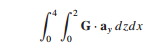### Create an Account

Already have account?

### Forgot Your Password ?

Home / Questions / A field is given as G 25x2 yxax yay Find (a) a unit vector in the direction of G at

# A field is given as G 25x2 yxax yay Find (a) a unit vector in the direction of G at

A field is given as G = [25/(x2 + y2)](xax + yay ). Find (a) a unit vector in the direction of G at P(3, 4, −2); (b) the angle between G and ax at P; (c) the value of the following double integral on the plane y = 7.2. By expressing diagonals as vectors and using the definition of the dot product, find the smaller angle between any two diagonals of a cube, where each diagonal connects diametrically opposite corners and passes through the center of the cube.

Jun 14 2020 View more View Less

#### Answer (Solved)Subscribe To Get Solution